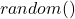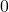# C Program to Generate Random Numbers

C language has a built-in function to generate random numbers. In this program, we will use that function to generate a few random numbers.

Before you begin with the example, learn about C concepts. If you know the basics skip the step and continue with the example.

## Problem Definition

The program uses thefunction from C standard library and generates random numbers. Its only requirement is a max limit to the random numbers generated. Therefore, the random number is betweento max which is supplied by the user. Also, we keep thefunction inside of a loop so that this process is repeated for n times. The value of n is input by the user.

## Program Source Code

#include <stdio.h>
#include <stdlib.h>

int main()
{

int n, max, num, c;

printf("Enter Number of Random Numbers You Want:");
scanf("%d", &n);

printf("Enter Maximum value for the Random Numbers:");
scanf("%d", &max);

printf("%d random numbers from 0 to %d\n\n",n,max);

for(c = 1; c <= n; c++)
{

num = rand() % max + 1;
printf("%d\n",num);

}

system("PAUSE");
return 0;

}

## Output

Enter Number of Random Numbers You Want: 10
Enter Maximum value for the Random Numbers: 1000
10 random numbers from 0 to 1000

42
468
335
501
170
725
479
359
963
465
Press any key to continue . . . _